# SquareCirclez - the IntMath blog

## Latest articles...

### How to find the equation of a logarithm function from its graph?

29 May 2019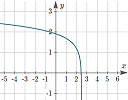This article explains how to determine the equation of a logarithmic function given its graph

### How to search for solutions to math questions

24 April 2019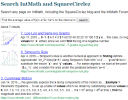A lot of students struggle to find answers for their math questions. Here are some helpful hints.

### Units: Calories and kilograms

14 December 2018How many calories? and the way the kilogram was defined has been in place since 1799. It recently got a makeover.

### Spiral around a hyperboloid - the Effekt bridge

26 August 2018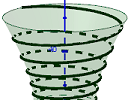We explore how to find the equations for a spiral around a hyperboloid.

### Infinite series background

28 June 2018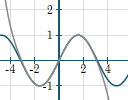Infinite series have played an important role in the development of mathematics, especially calculus. Here's some background to some applets I wrote recently.

### The object with finite volume but infinite surface area

22 March 2018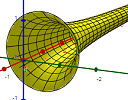This object has a weird property - it has finite volume, but infinite surface area. How is this possible?

### Double springs interactive graph

25 October 2017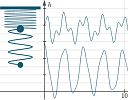The revised double spring applet now allows exploration on mobile devices. Here is some background.

### Frequency of notes on a piano - interactive learning object

31 July 2017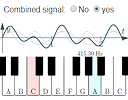Explore piano note frequencies in this interactive learning object, combining trig graphs and exponential growth.

### New applet: What does b do in a quadratic function?

30 June 2017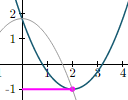y = ax2 + bx + c is a parabola. Changing the variables a and c are fairly simple, but what does b actually change, and by how much?

### New applet: Domain and range investigation

9 May 2017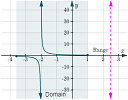This interactive applet allows you to explore the concepts of domain and range for several different mathematical functions.